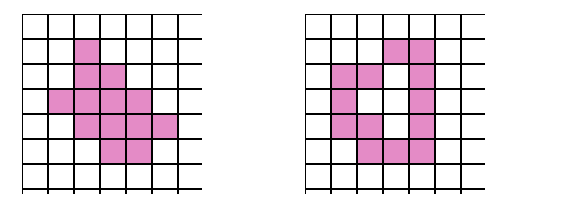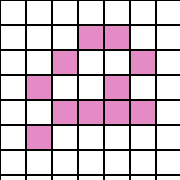Defining Connectivity

Introduction

In binary valued digital imaging, a pixel can either have a value of  1 -when it's part of the pattern- , or  0 -when it's part of the background- i.e. there is no grayscale level. (We will assume that pixels with value 1 are black while zero valued pixels are white).

In order to identify objects in a digital pattern, we need to locate groups of black pixels that are "connected" to each other. In other words, the objects in a given digital pattern are the connected components of that pattern.

In general, a connected component is a set of black pixels, P, such that for every pair of pixels pi and pj in P, there exists a sequence of pixels  pi, ..., pj   such that:
a) all pixels in the sequence are in the set P i.e. are black, and
b) every 2 pixels that are adjacent in the sequence are "neighbors"

As a result, an important question arises: When can we say that 2 pixels are "neighbors"?
Since we are using square pixels, the answer to the previous question is not trivial. The reason for that is: in a square tessellation, pixels either share an edge, a vertex, or neither. There are 8 pixels sharing an edge or a vertex with any given pixel; these pixels make up the Moore neighborhood of that pixel. Should we consider pixels having only a common vertex as "neighbors" ? Or should 2 pixels have a common edge in order for them to be considered  "neighbors"?

This gives rise to 2 types of connectedness, namely: 4-connectivity and 8-connectivity.

4-Connectivity

When can we say that a given set of black pixels is 4-connected ?
First, we have to define the concept of a 4-neighbor (also known as a direct-neighbor):

Definition of a 4-neighbor:
A pixel, Q, is a 4-neighbor  of a given pixel, P, if Q and P share an edge.
The 4-neighbors of pixel P (namely pixels P2,P4,P6 and P8) are shown in Figure 2 below.Definition of a 4-connected component :
A set of black pixels, P, is a 4-connected component if for every pair of pixels pi and pj in P, there exists a sequence of pixels  pi, ..., pj such that:
a) all pixels in the sequence are in the set P i.e. are black, and
b) every 2 pixels that are adjacent in the sequence are 4-neighbors

Examples of 4-connected patterns :
The following diagrams are examples of patterns that are 4-connected:8-Connectivity

When can we say that a given set of black pixels is 8-connected ?
First, we have to define the concept of an 8-neighbor (also known as an  indirect-neighbor):

Definition of an 8-neighbor:
A pixel, Q, is an 8-neighbor (or simply a neighbor) of a given pixel, P, if Q and P either share an edge or a vertex.
The 8-neighbors of a given pixel P make up the Moore neighborhood of that pixel.

Definition of an 8-connected component:
A set of black pixels, P, is an 8-connected component (or simply a connected component) if for every pair of pixels pi and pj in P, there exists a sequence of pixels pi, ..., psuch that:
a) all pixels in the sequence are in the set P i.e. are black, and
b) every 2 pixels that are adjacent in the sequence are 8-neighbors

NOTE
All 4-connected patterns are 8-connected i.e. 4-connected patterns are a subset of the set of 8-connected patterns.
On the other hand, an 8-connected pattern may not be 4-connected.

Example of 8-connected pattern :
The diagram below is an example of a pattern that is 8-connected but not 4-connected:Example of a pattern that's not 8-connected:
The diagram below is an example of a pattern that is not 8-connected i.e. is made up of more than one connected component (there are 3 connected components in the diagram below):All comments and questions are welcomed...
abeer.ghuneim@mail.mcgill.ca